# 瞭解 Transformers 是如何“思考”的

Transformer 模型是 AI 系統的基礎。已經有了數不清的關於 "Transformer 如何工作" 的核心結構圖表。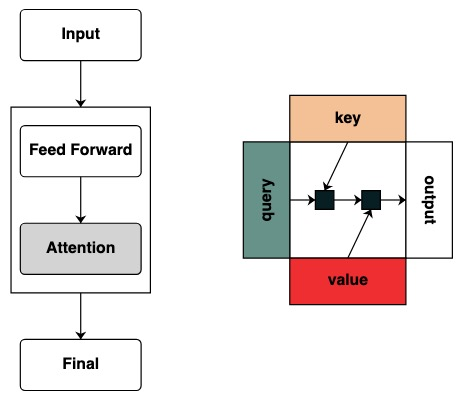Thinking Like Transformers 這篇論文中提出了 transformer 類的計算框架，這個框架直接計算和模仿 Transformer 計算。使用 RASP 程式語言，使每個程式編譯成一個特殊的 Transformer。

``````!pip install git+https://github.com/srush/RASPy
``````

``````def flip():
length = (key(1) == query(1)).value(1)
flip = (key(length - indices - 1) == query(indices)).value(tokens)
return flip
flip()
``````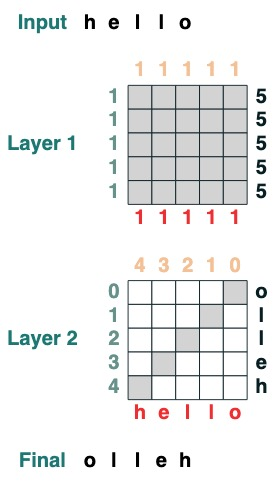## 文章目錄

• 第一部分：Transformers 作為程式碼
• 第二部分：用 Transformers 編寫程式

## Transformers 作為程式碼

### 輸入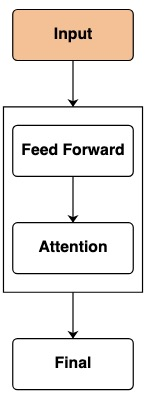``````tokens
``````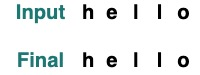``````tokens.input([5, 2, 4, 5, 2, 2])
``````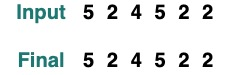``````indices
``````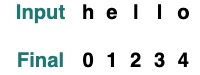``````sop = indices
sop.input("goodbye")
``````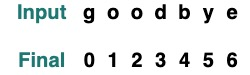### 前饋網路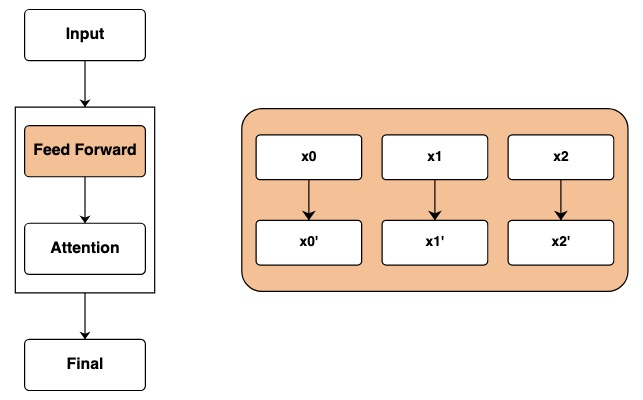``````tokens == "l"
``````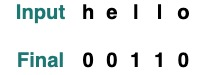``````model = tokens * 2 - 1
model.input([1, 2, 3, 5, 2])
``````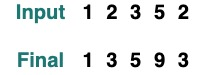``````model = tokens - 5 + indices
model.input([1, 2, 3, 5, 2])
``````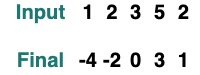``````(tokens == "l") | (indices == 1)
``````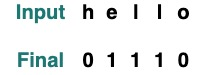``````where((tokens == "h") | (tokens == "l"), tokens, "q")
``````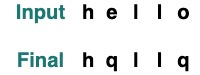`map` 使我們可以定義自己的操作，例如一個字串以 `int` 轉換。（使用者應謹慎使用可以使用的簡單神經網路計算的操作）

``````atoi = tokens.map(lambda x: ord(x) - ord('0'))
atoi.input("31234")
``````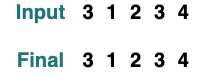``````def atoi(seq=tokens):
return seq.map(lambda x: ord(x) - ord('0'))

op = (atoi(where(tokens == "-", "0", tokens)) + 2)
op.input("02-13")
``````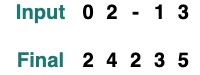### 注意力篩選器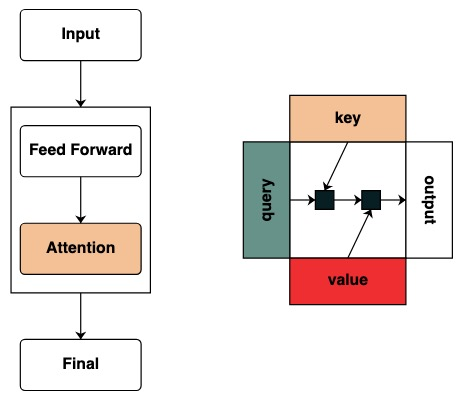``````key(tokens)
``````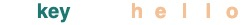``````query(tokens)
``````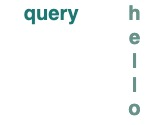``````query(1)
``````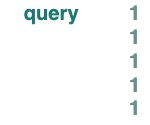``````eq = (key(tokens) == query(tokens))
eq
``````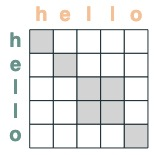• 選擇器的匹配位置偏移 1:
``````offset = (key(indices) == query(indices - 1))
offset
``````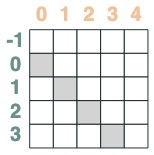• key 早於 query 的選擇器:
``````before = key(indices) < query(indices)
before
``````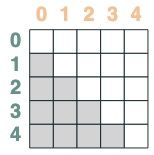• key 晚於 query 的選擇器:
``````after = key(indices) > query(indices)
after
``````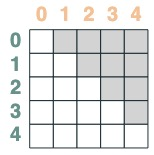``````before & eq
``````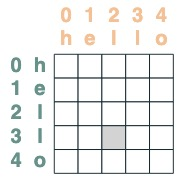## 使用注意力機制

(請注意：在原始論文中，他們使用一個平均聚合操作並且展示了一個巧妙的結構，其中平均聚合能夠代表總和計算。RASPy 預設情況下使用累加來使其簡單化並避免碎片化。實際上，這意味著 raspy 可能低估了所需要的層數。基於平均值的模型可能需要這個層數的兩倍)

``````(key(tokens) == query(tokens)).value(1)
``````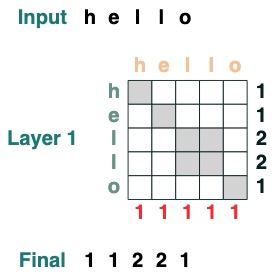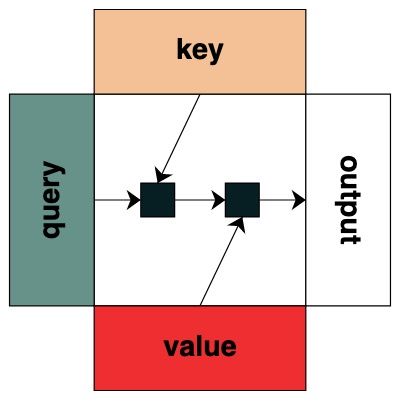``````length = (key(1) == query(1)).value(1)
length = length.name("length")
length
``````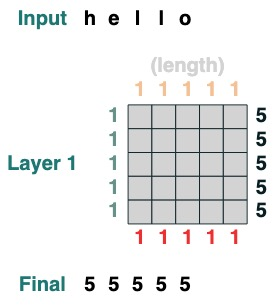``````WINDOW=3
s1 = (key(indices) >= query(indices - WINDOW + 1))
s1
``````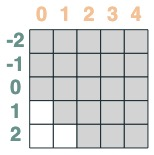``````s2 = (key(indices) <= query(indices))
s2
``````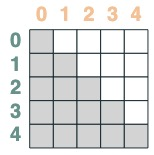``````sel = s1 & s2
sel
``````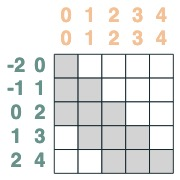``````sum2 = sel.value(tokens)
sum2.input([1,3,2,2,2])
``````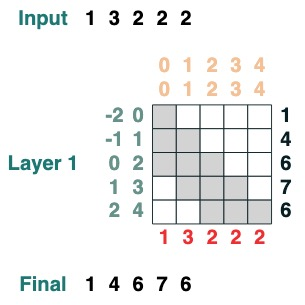``````def cumsum(seq=tokens):
x = (before | (key(indices) == query(indices))).value(seq)
return x.name("cumsum")
cumsum().input([3, 1, -2, 3, 1])
``````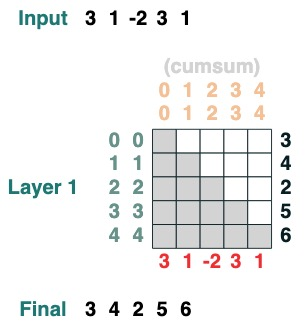### 層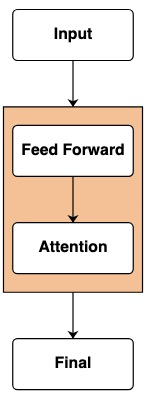``````x = cumsum(length - indices)
x.input([3, 2, 3, 5])
``````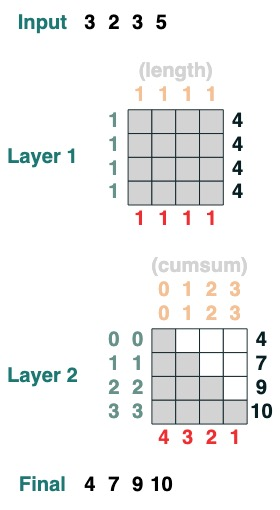## 用 transformers 進行程式設計

### 挑戰一：選擇一個給定的索引

``````def index(i, seq=tokens):
x = (key(indices) == query(i)).value(seq)
return x.name("index")
index(1)
``````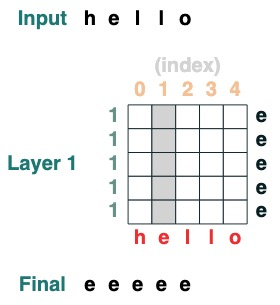### 挑戰二：轉換

``````def shift(i=1, default="_", seq=tokens):
x = (key(indices) == query(indices-i)).value(seq, default)
return x.name("shift")
shift(2)
``````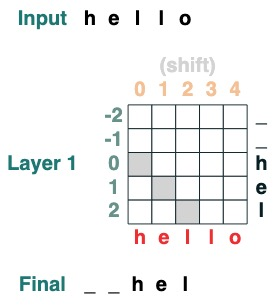### 挑戰三：最小化

``````def minimum(seq=tokens):
sel1 = before & (key(seq) == query(seq))
sel2 = key(seq) < query(seq)
less = (sel1 | sel2).value(1)
x = (key(less) == query(0)).value(seq)
return x.name("min")
minimum()([5,3,2,5,2])
``````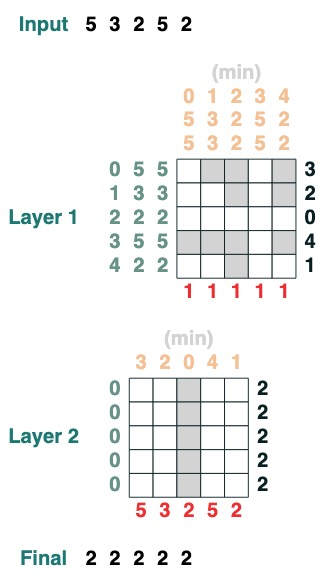### 挑戰四：第一索引

``````def first(q, seq=tokens):
return minimum(where(seq == q, indices, 99))
first("l")
``````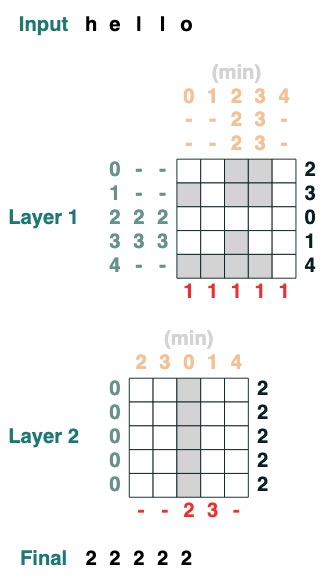### 挑戰五：右對齊

``````def ralign(default="-", sop=tokens):
c = (key(sop) == query("_")).value(1)
x = (key(indices + c) == query(indices)).value(sop, default)
return x.name("ralign")
ralign()("xyz__")
``````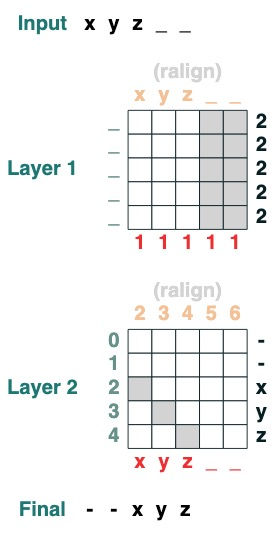### 挑戰六：分離

``````def split(v, i, sop=tokens):

mid = (key(sop) == query(v)).value(indices)
if i == 0:
x = ralign("0", where(indices < mid, sop, "_"))
return x
else:
x = where(indices > mid, sop, "0")
return x
split("+", 1)("xyz+zyr")
``````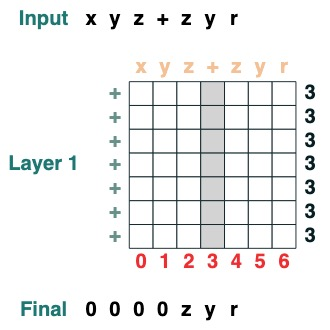``````split("+", 0)("xyz+zyr")
``````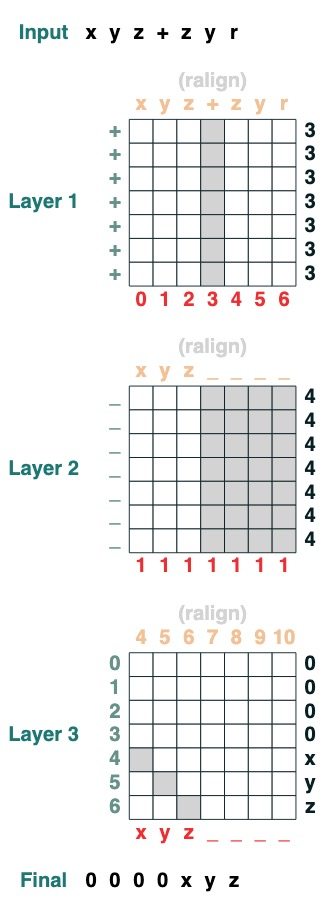### 挑戰七：滑動

``````def slide(match, seq=tokens):
x = cumsum(match)
y = ((key(x) == query(x + 1)) & (key(match) == query(True))).value(seq)
seq =  where(match, seq, y)
return seq.name("slide")
slide(tokens != "<").input("xxxh<<<l")
``````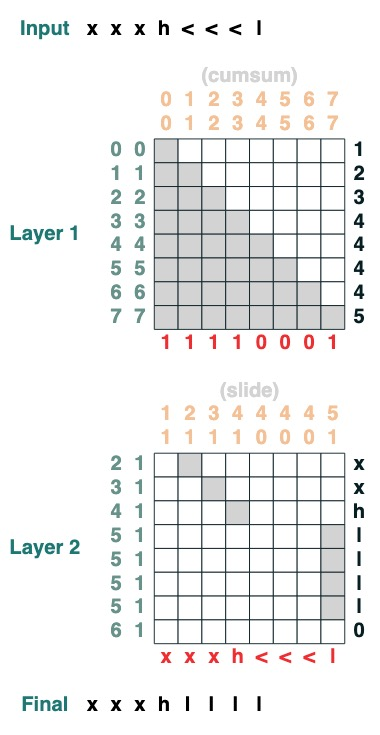## 挑戰八：增加

``````add().input("683+345")
``````
1. 分成兩部分。轉製成整形。加入

“683+345” => [0, 0, 0, 9, 12, 8]

1. 計算攜帶條款。三種可能性：1 個攜帶，0 不攜帶，< 也許有攜帶。

[0, 0, 0, 9, 12, 8] => “00<100”

1. 滑動進位係數

“00<100” => 001100"

1. 完成加法

``````def add(sop=tokens):
x = atoi(split("+", 0, sop)) + atoi(split("+", 1, sop))
# 1) Check for carries
carry = shift(-1, "0", where(x > 9, "1", where(x == 9, "<", "0")))
# 2) In parallel, slide carries to their column
carries = atoi(slide(carry != "<", carry))
return (x + carries) % 10
``````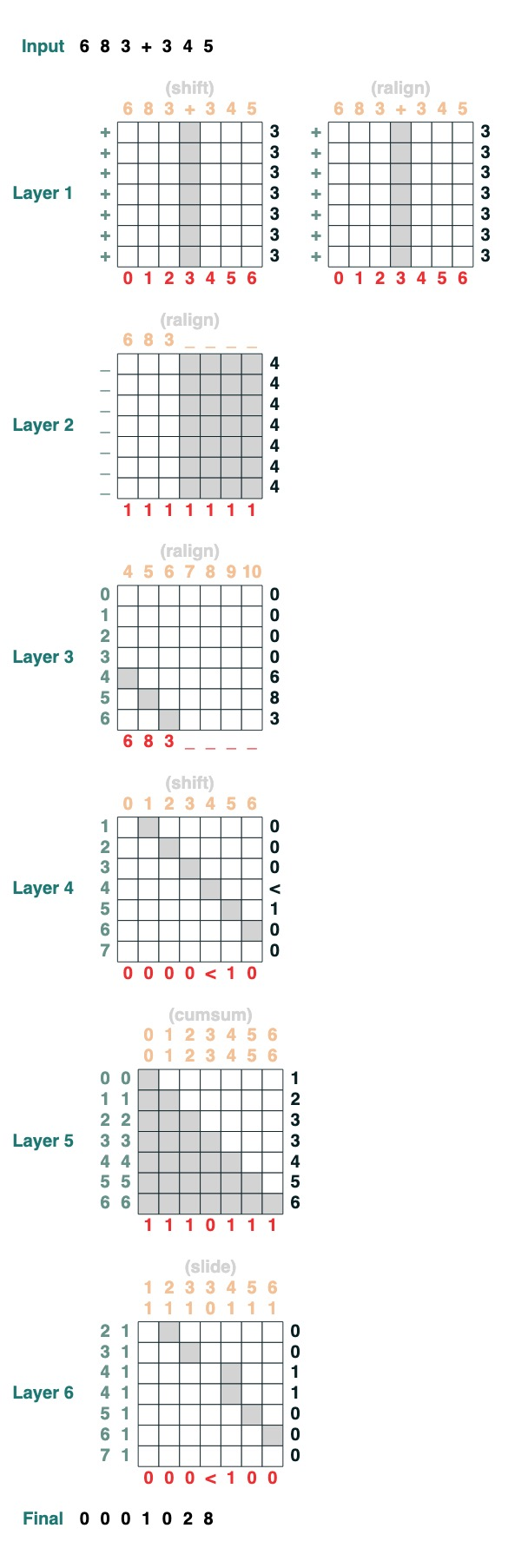``````683 + 345
``````
``````1028
``````

<hr>